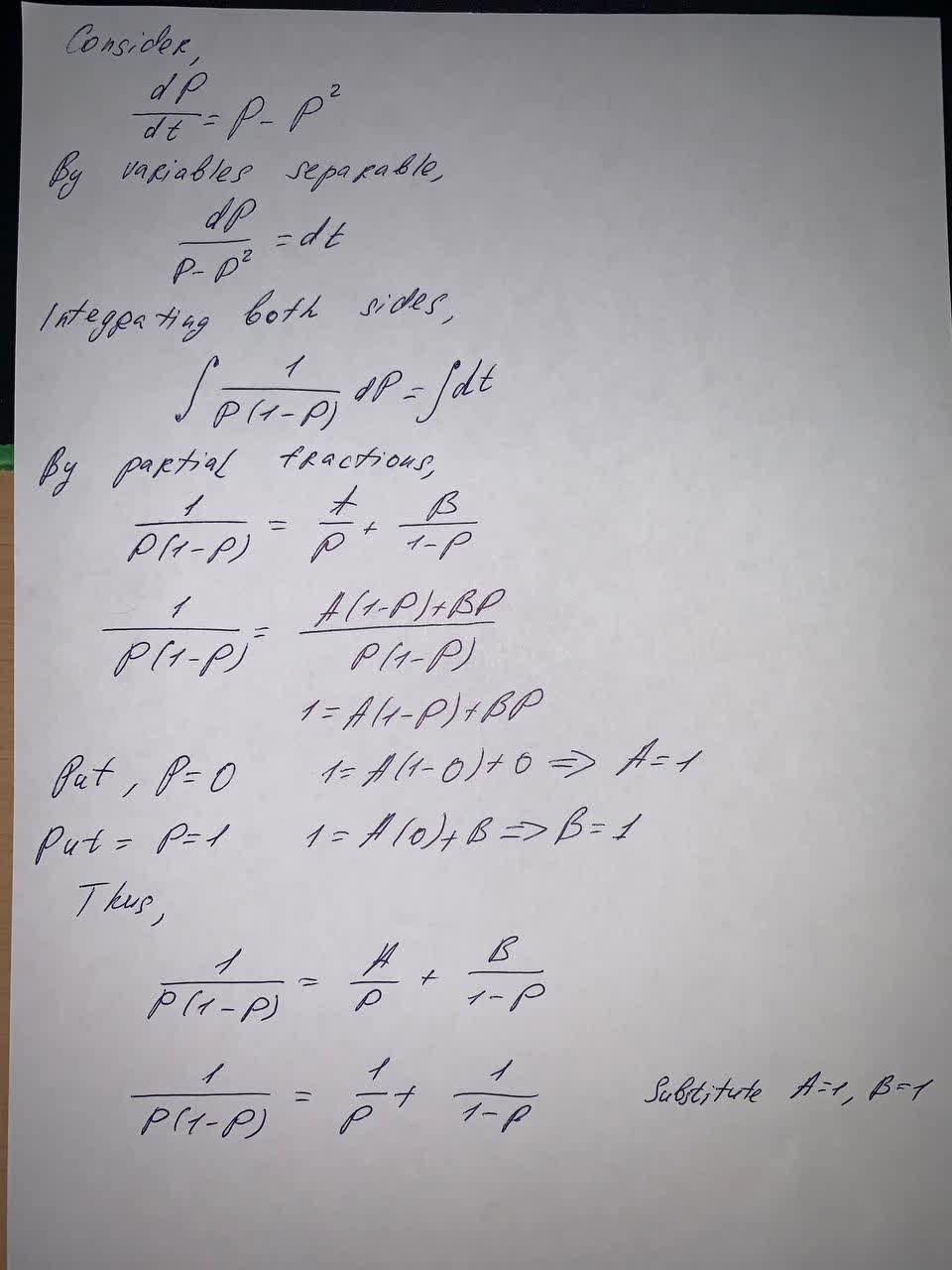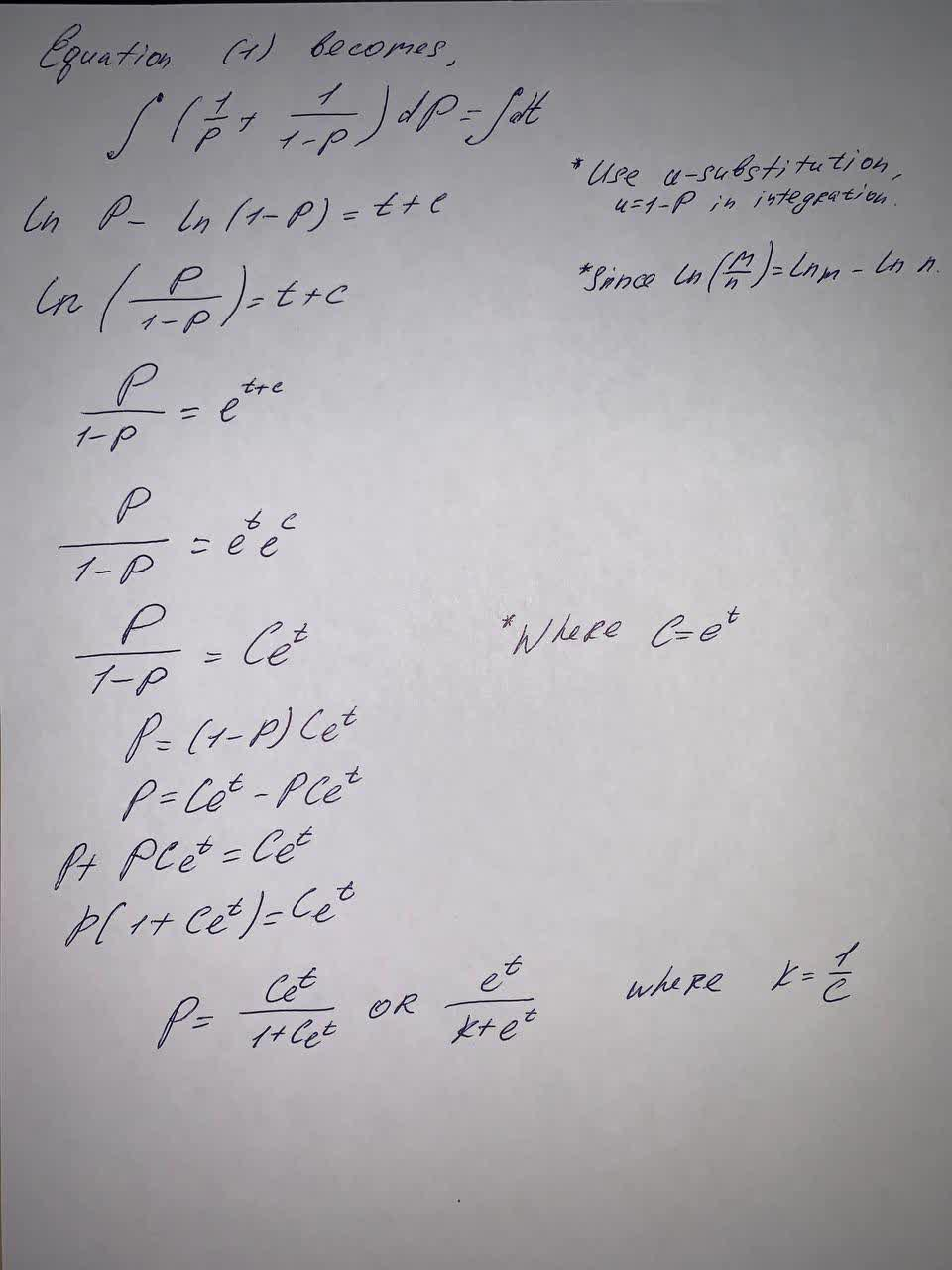# Solve the given differential equation by separation of variables: \frac{dP}{dt}=PTabansi 2021-09-15 Answered
Solve the given differential equation by separation of variables:
$$\displaystyle{\frac{{{d}{P}}}{{{\left.{d}{t}\right.}}}}={P}-{P}^{{2}}$$

• Questions are typically answered in as fast as 30 minutes

### Plainmath recommends

• Get a detailed answer even on the hardest topics.
• Ask an expert for a step-by-step guidance to learn to do it yourself.StrycharzT
To solve: